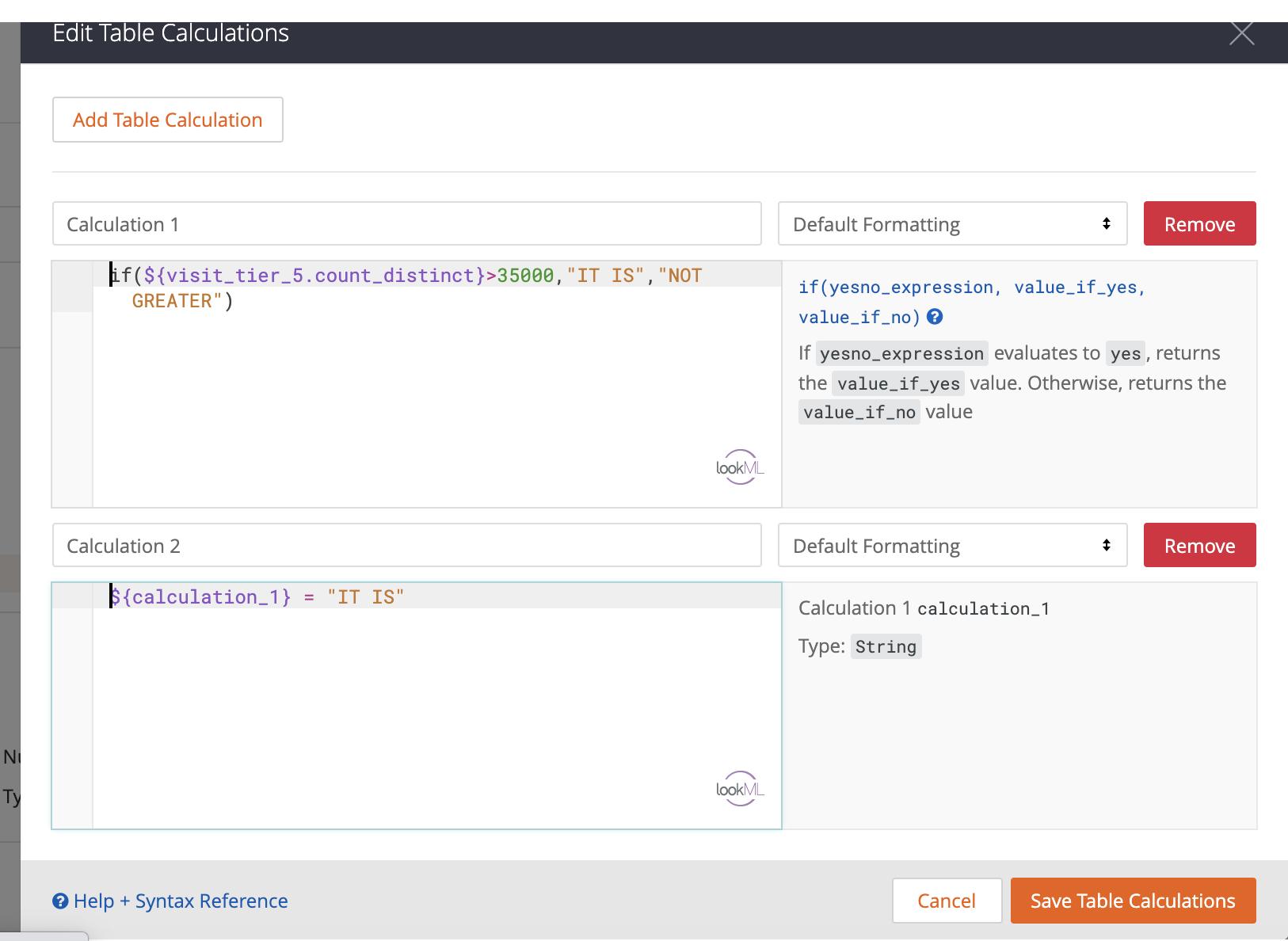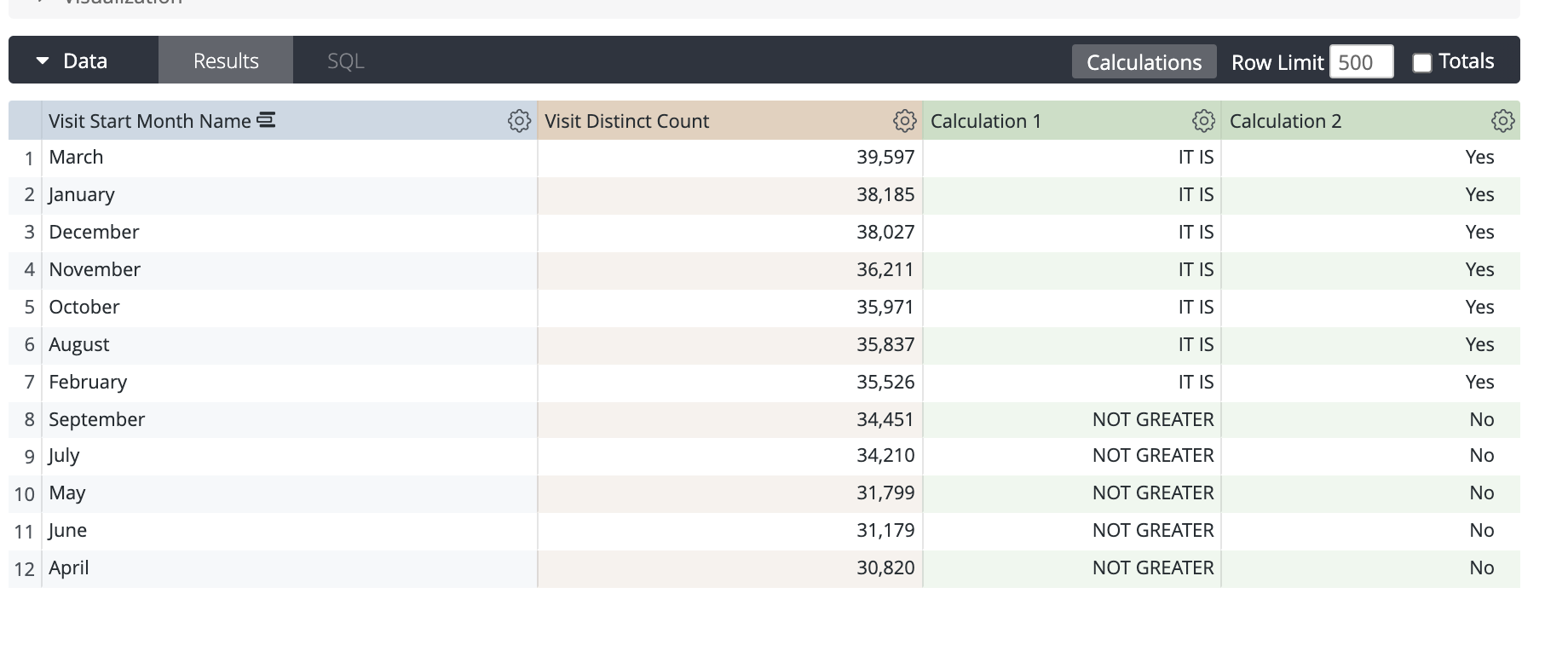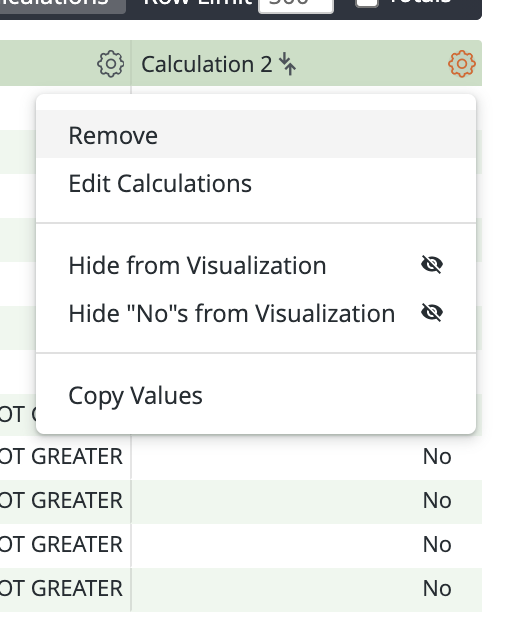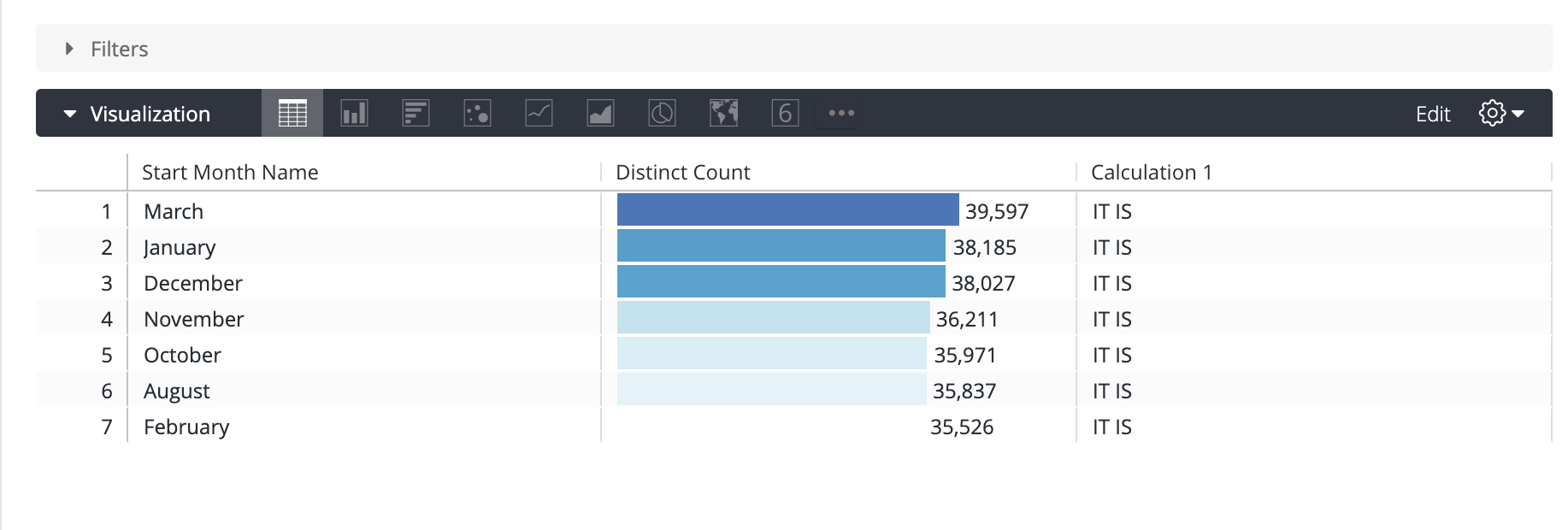• Official commentHi Jan,

This is possible but you would need to create another condition to the calculation. For example, if you created "Calculation 1"  and one of the outputs is 1000, you can create another calculation "Calculation 2" with the pure value you wish to filter on, for example, where Calculation 1 = 1000. That will give you a yes / no answer, if you click the gear icon, it will show the option to hide No's from Visualization.•Worked perfectly! (I did see this in the looker instructions but couldn't figure out the 2nd calculation)

Thank you.

•Great! Thanks for letting us know Jan :)

Alex